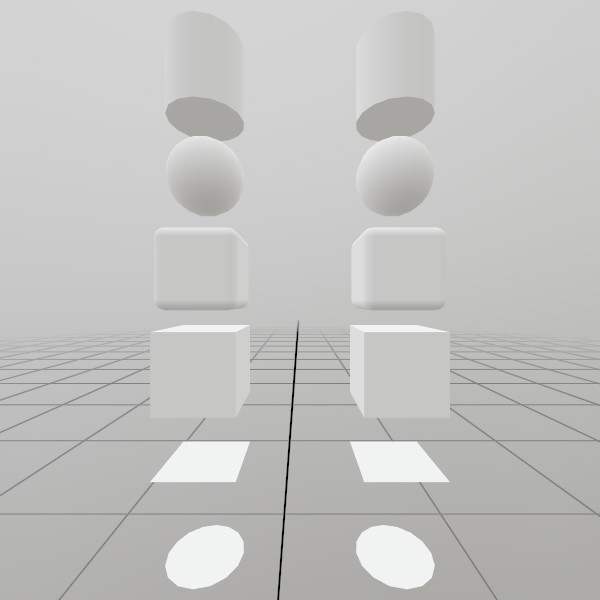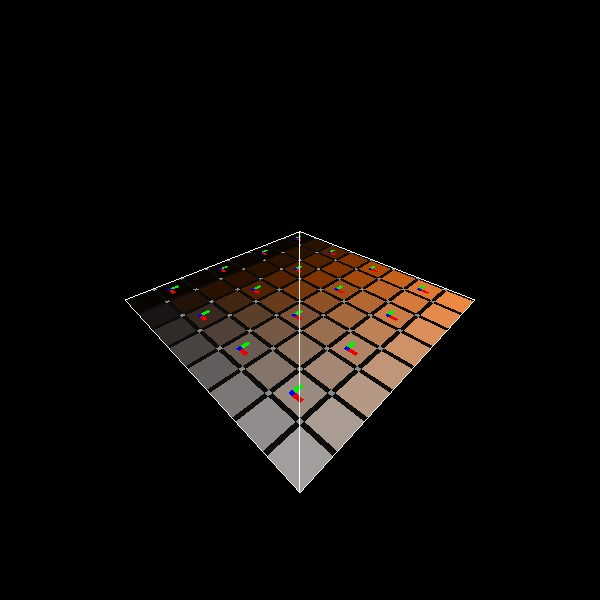# Mesh.GeneratePlane

``````static Mesh GeneratePlane(Vec2 dimensions, int subdivisions)
``````

Generates a plane on the XZ axis facing up that is optionally subdivided, pre-sized to the given dimensions. UV coordinates start at 0,0 at the -X,-Z corer, and go to 1,1 at the +X,+Z corner!

NOTE: This generates a completely new Mesh asset on the GPU, and is best done during ‘initialization’ of your app/scene. You may also be interested in using the pre-generated `Mesh.Quad` asset if it already meets your needs.

Vec2 dimensions How large is this plane on the XZ axis, in meters?
int subdivisions Use this to add extra slices of vertices across the plane. This can be useful for some types of vertex-based effects!
RETURNS: Mesh A plane mesh, pre-sized to the given dimensions.
``````static Mesh GeneratePlane(Vec2 dimensions, Vec3 planeNormal, Vec3 planeTopDirection, int subdivisions)
``````

Generates a plane with an arbitrary orientation that is optionally subdivided, pre-sized to the given dimensions. UV coordinates start at the top left indicated with ‘planeTopDirection’.

NOTE: This generates a completely new Mesh asset on the GPU, and is best done during ‘initialization’ of your app/scene. You may also be interested in using the pre-generated `Mesh.Quad` asset if it already meets your needs.

Vec2 dimensions How large is this plane on the XZ axis, in meters?
Vec3 planeNormal What is the normal of the surface this plane is generated on?
Vec3 planeTopDirection A normal defines the plane, but this is technically a rectangle on the plane. So which direction is up? It’s important for UVs, but doesn’t need to be exact. This function takes the planeNormal as law, and uses this vector to find the right and up vectors via cross-products.
int subdivisions Use this to add extra slices of vertices across the plane. This can be useful for some types of vertex-based effects!
RETURNS: Mesh A plane mesh, pre-sized to the given dimensions.

## Examples

### Generating a Mesh and ModelHere’s a quick example of generating a mesh! You can store it in just a Mesh, or you can attach it to a Model for easier rendering later on.

``````// Do this in your initialization
Mesh  planeMesh  = Mesh.GeneratePlane(Vec2.One*0.4f);
Model planeModel = Model.FromMesh(planeMesh, Default.Material);
``````

Drawing both a Mesh and a Model generated this way is reasonably simple, here’s a short example! For the Mesh, you’ll need to create your own material, we just loaded up the default Material here.

``````Matrix planeTransform = Matrix.T(-.5f, -1, 0);
planeMesh.Draw(Default.Material, planeTransform);

planeTransform = Matrix.T(.5f, -1, 0);
planeModel.Draw(planeTransform);
``````

### UV and Face layout

Here’s a test image that illustrates how this mesh’s geometry is laid out.``````meshPlane = Mesh.GeneratePlane(Vec2.One);
``````

Found an issue with these docs, or have some additional questions? Create an Issue on Github!